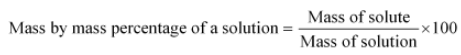# Insulin contains 3.4% Sulphur. What is the minimum molecular weight oh insulin?

Insulin contains 3.4% Sulphur. What is the minimum molecular weight oh insulin?

In this case, the data is insufficient. The number of sulphur atoms present in the Insuline is not given in the question.
Therefore, the minimum number of sulphur atoms that can be present in this molecule is one.
The molar mass of sulphur = 32 g per mole.
Let x grams be the molar mass of Insulin. The mass by mass percentage is given by,We are given that the percentage of sulphur is 3.4 %. So
3.4 = (32 / x) × 100
Therefore, 3.4 x = 32 × 100
x = 941.176 g.
Hence, the minimum molecular weight of Insulin is 941.176 g.